# In Each of the Following, Draw Perpendicular Through Point P to the Line Segment Ab : - Mathematics

Sum

In each of the following, draw perpendicular through point P to the line segment AB :

(i)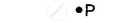(ii)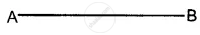(iii)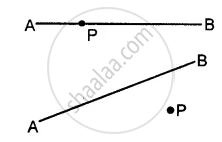#### Solution

(i) Steps of Construction :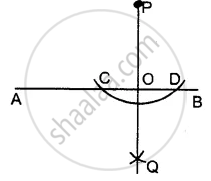1. With P as a centre, draw an arc of a suitable radius which cuts AB at points C and D.
2. With C and D as centres, draw arcs of equal radii and let these arcs intersect each other at point Q.
[The radius of these arcs must be more than half of CD and both the arcs must be drawn on the other side]
3. Join P and Q
4. Let PQ cut AB at the point O.
Thus, OP is the required perpendicular clearly, ∠AOP = ∠BOP = 90°

(ii) Steps of Construction :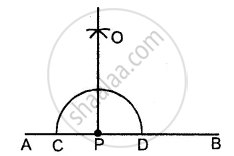1. With P as a centre, draw an arc of any suitable radius which cuts AB at points C and D.
2. With C and D as centres, draw arcs of equal radii. Which intersect each other at point A.
[This radius must be more than half of CD and let these arc intersect each other at the point 0]
3. Join P and O. Then OP is the required perpendicular.
∠OPA = ∠OPB = 90°

(iii) Steps of Construction :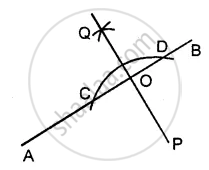1. With P as a centre, draw an arc of any suitable radius which cuts AB at points C and D.
2. With C and D as a centre, draw arcs of equal radii
[The radius of these arcs must be more than half of CD and both the arcs must be drawn on the other side.]
and let these arcs intersect each other at the point Q.
3. Join Q and P. Let QP cut AB at the point O. Then OP is the required perpendicular.
Clearly, ∠AOP = ∠BOP = 90°
Concept: Drawing the Perpendicular Bisector of a Line Segment
Is there an error in this question or solution?

#### APPEARS IN

Selina Concise Mathematics Class 8 ICSE
Chapter 18 Constructions
Exercise 18 (B) | Q 3 | Page 204